## LetsPlayMaths.Com

WELCOME TO THE WORLD OF MATHEMATICS

# Class-7 Profit and Loss

Introduction to Profit and Loss

Cost Price

Selling Price

Profit

Loss

Profit Percent

Loss Percent

Formulae to Remember

Profit And Loss Worksheet

## Introduction to Profit and Loss

In our daily life, consumer buy various articles from the shopkeepers. These articles are either directly bought from manufacturers or through wholesalers. Shopkeepers sell these articles higher than the rate at which they bought them to earn money.

## Cost Price

The money paid by the shopkeeper to buy the goods from manufacturer or wholesaler is known as Cost Price (C.P).

## Selling Price

The price at which a shopkeeper sells the goods/products is known as Selling Price (S.P).

## Profit

If the selling price (S.P) of a goods is greater than the cost price (C.P), then the shopkeeper makes profit / gain.

Profit = Selling Price − Cost Price

Let's see some examples.

Example 1. Cost price of a dress is Rs. 500 and selling price of it is Rs. 650. Find the amount of profit made by the shopkeeper.

Solution. Cost Price = Rs. 500

Selling Price = Rs. 650

Profit = Selling Price − Cost Price

= 650 − 500

= Rs. 150

Hence, the profit made by the shopkeeper is equal to Rs. 150

Example 2. Selling price of an article is Rs. 2460 and profit made by the shopkeeper is Rs. 120.50. Find the cost price of the article.

Solution. Profit = Selling Price − Cost Price

=> Cost Price = Selling Price − Profit

Cost Price = Rs. 2460 − Rs. 120.50

= Rs. 2339.50

Hence, cost price of the article is Rs. 2339.50.

Example 3. Cost price of an article is Rs. 98. If the shopkeeper sells the article with a profit of Rs. 27, then what is the selling price?

Solution. Profit = Selling Price − Cost Price

=> Selling Price = Cost Price + Profit

= Rs. 98 + Rs. 27

= Rs. 125

Hence, selling price of the article is Rs. 125.

## Loss

If the selling price (S.P) of a goods is less than the cost price (C.P), then the shopkeeper makes a loss.

Loss = Cost Price − Selling Price

Let's see some examples.

Example 1. If cost price of an article is 725 and selling price of it is Rs. 715, then find the loss incurred by the shopkeeper.

Solution. Loss = Cost Price − Selling Price

= Rs. 725 − Rs. 715

= Rs. 10

Hence, loss incurred by the shopkeeper is Rs. 10.

Example 2. One man bought a cycle for Rs. 3780. He used it for 1 year, then sold it at a loss of Rs. 750. Find the selling price of the cycle.

Solution. Cost price of the cycle = Rs. 3780

Loss incurred by the man = Rs. 750

Selling Price = Cost Price − Loss

= Rs. 3780 − Rs. 750

= Rs. 3030

Hence, selling price of the cycle is Rs. 3030.

Example 3. An article was sold for Rs. 400 at a loss of Rs. 72. Find the cost price of the article.

Solution. Loss = Cost Price − Selling Price

=> Cost Price = Selling Price + Loss

= Rs. 400 + Rs. 72

= Rs. 472

Hence, cost price of the article is Rs. 472.

## Profit Percent

The profit percentage is the profit that would be obtained for a cost price of Rs. 100.

Profit percent = ProfitCost Price × 100

Let's see some examples.

Example 1. If cost price of an article is Rs. 500 and selling price is Rs. 575, then find the profit percentage.

Solution. Cost Price = Rs. 500

Selling Price = Rs. 575

Profit = Rs. 575 − Rs. 500

= Rs. 75

Profit Percentage = ProfitCost Price × 100

= 75500 × 100 = 15%

Hence, the profit percentage is 15%

Example 2. Maria bought apples at Rs. 120 per kg. If she had to sell them at a profit of 20%, then find the selling price.

Solution. Cost price of apple per kg = Rs. 120

Profit Percentage = ProfitCost Price × 100

=> 20 = Profit120 × 100

=> Profit = (20×120)100

=> Profit = Rs. 24

Selling Price = Cost Price + Profit

= Rs. 120 + Rs. 24

= Rs. 144

Hence, the selling price is equal to Rs. 144.

Example 3. A shopkeeper sells his product at a profit of 12%. If his selling price is Rs. 95984, then find the cost price.

Solution. Let's assume cost price to be 'p'.

Profit = 12% of p = 12p100

p = 95984 − 12p100

=> p + 12p100 = 95984

=> (100p + 12p)100 = 95984

=> 112p = 95984 × 100

=> p = (95984 × 100)112

=> p = 85700

Hence, cost price of the product is Rs. 85700

## Loss Percent

The loss percentage is the loss that would be obtained for a cost price of Rs. 100.

Loss percent = LossCost Price × 100

Let's see some examples.

Example 1. A chair was bought for Rs. 800 and sold for Rs. 736. Find the loss and loss percentage.

Solution. Cost price of the chair = Rs. 800

Selling price of the chair = Rs. 736

Here selling price is less than cost price, so shopkeeper incurred a loss.

Loss = Cost Price − Selling Price

= Rs. 800 − Rs. 736

= Rs. 64

Loss Percentage = 64800 × 100 = 8%

Hence, loss is equal to Rs. 64 and percentage of loss is equal to 8%.

Example 2. Find the selling price of a wall clock whose cost price is Rs. 650 and loss percentage is 6%.

Solution. Cost price of wall clock = Rs. 650

Let's assume loss = L

Loss percent = LossCost Price × 100

=> 6 = L650 × 100

=> L = (650×6)100

=> L = Rs. 39

Selling Price = Cost Price − Loss

= Rs. 650 − Rs. 39

= Rs. 611

Hence, selling price of the wall clock is Rs. 611.

Example 3. By selling a motorbike for Rs. 92,340, Mr. Mishra losses 10%. For how much should he sell it to gain 12%.

Solution. Selling Price = Rs. 92,340

Loss = 10%

CP = {100(100−Loss%) × SP}

= 100(100−10) × 92340

= 10090 × 92340

= Rs. 102600

In order to make 12% profit selling price would be

= CP + (12% of CP)

= 102600 + 102600 × 12100

= Rs. 114912

Hence, the selling price of the motorbike should be Rs. 114912.

## Formulae to Remember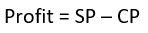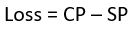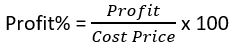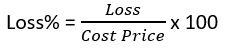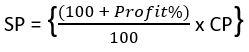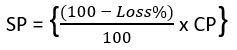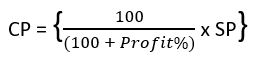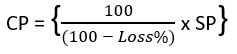## Profit And Loss Worksheet

Profit & Loss Worksheet - 1

Profit & Loss Worksheet - 2